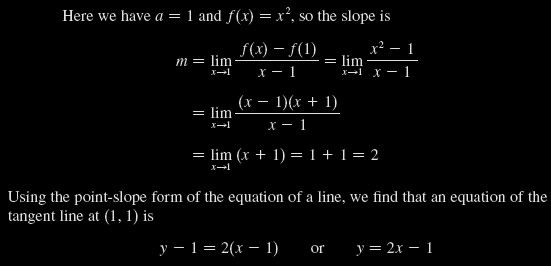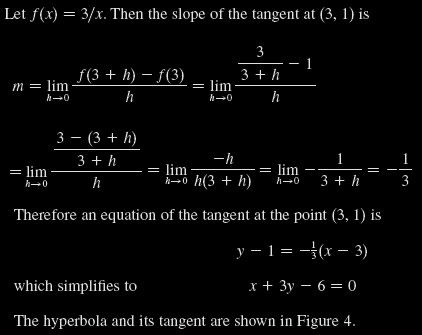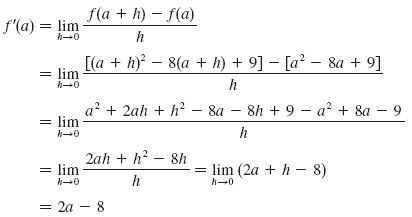# Stewart – Calculus – 2.7 – Derivatives and Rates of Change

1. Find an equation of the tangent line to the parabola$y=x^{2}$ at the point$P(1,1)$.
2. Find an equation of the tangent line to the hyperbola$f(x)=\frac{3}{x}$ at the point$(3, 1)$.
3. Find the derivative of the function$f(x)=x^{2}-8x+9$ at the number$a$.